Electron. J. Diff. Equ., Vol. 2016 (2016), No. 227, pp. 1-9.

### Existence and nonexistence of solutions for semilinear equations on exterior domains Joseph A. Iaia

Abstract:
In this article we study radial solutions of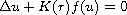on the exterior of the ball of radius R>0 centered at the origin in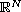where f is odd with f<0 on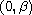, f>0 on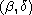,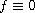for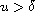, and where the function K(r) is assumed to be positive and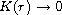as. The primitive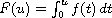has a "hilltop" at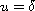. We prove that if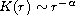with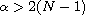and if R>0 is sufficiently small then there are a finite number of solutions ofon the exterior of the ball of radius R such that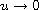as. We also prove the nonexistence of solutions if R is sufficiently large.

Submitted July 20, 2016. Published August 22, 2016.
Math Subject Classifications: 34B40, 35B05.
Key Words: Exterior domains; semilinear; superlinear; radial.

Show me the PDF file (238 KB), TEX file for this article.Joseph A. Iaia Department of Mathematics University of North Texas P.O. Box 311430 Denton, TX 76203-1430, USA email: iaia@unt.edu